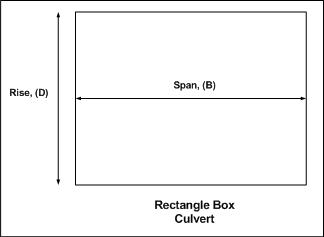* Log In to use the Calculate function * Become a Member!

Trial Design Culvert Selection - Box Culvert:

Calculation:
Designer/Checker:

Input:Maximum Allowable Headwater Depth (HWmax - ft or m)
Design flow/discharge (Q - cfs or cms)
Maximum Allowable Culvert Rise/Height (Dmax - ft or m)

Output:

Allowable Head (Hal) = HWmax - Dmax/2 =
Required Cross Section Area (AR) = 0.45Q/Hal^0.5 =
Total Width of Cross Section (W) = AR/Dmax =

Input:
Largest Barrel Span (S - ft or m), for which W is a Multiple

Output: